# RD Sharma Solutions for Class 10 Maths Chapter 8 Quadratic Equations Exercise 8.13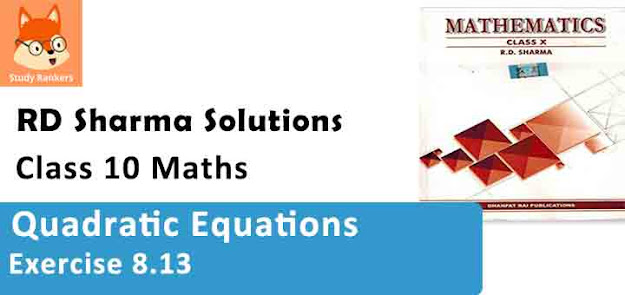1. A piece of cloth costs Rs. 35. If the piece were 4m longer and each metre costs Rs. one less, the cost would remain unchanged. How long is the piece ?

Solution

Let the length of piece of cloth = x m
Total cost = Rs. 35
Cost of 1 m cloth = Rs. 35/x
According to the question,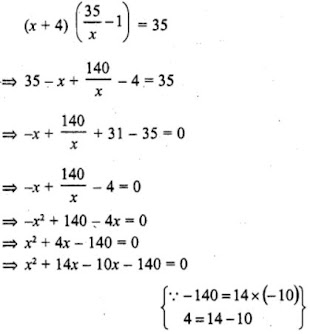⇒ x (x + 14) – 10 (x + 14) = 0
⇒ (x + 14) (x – 10) = 0
Either x + 14 = 0, then x = –14 which is not possible being negative
or x – 10 = 0, then x = 10
Length of piece of cloth = 10 m

2. Some students planned a picnic. The budget for food was Rs. 480. But eight of these failed to go and thus the cost of food for each member increased by Rs. 10. How many students attended the picnic ?

Solution

Let the number of students = x
and total budget = Rs. 480
Share of each students = Rs. 480/x
According to the question,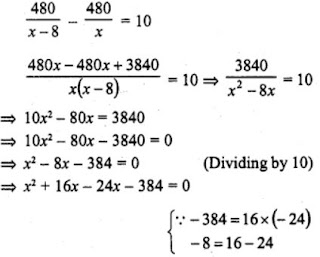⇒ x (x + 16) – 24 (x + 16) = 0
⇒ (x + 16) (x – 24) = 0
Either x + 16 = 0, then x = -16 which is not possible being negative
or x – 24 = 0, then x = 24
Number of students = 24
and number of students who attended the picnic = 24 – 8 = 16

3. A dealer sells an article for Rs. 24 and gains as much percent as the cost price of the article. Find the cost price of the article.

Solution

Let cost price ofjfie article = Rs. x
Selling price = Rs. 24
Gain = x %
According to the question,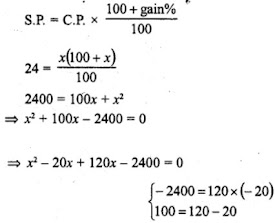⇒ x(x-20) + 120(x-20) = 0
⇒ (x-20) (x+120) = 0
Either x-20 = 0, then x = 20
or, x + 120 = 0, then x = -120 which is not possible as it is negative.
∴ Cost Price = Rs. 20

4. Out of a group of swans, 7/2 times the square root of the total number are playing on he share of a pond. The two remaining ones are swinging in water. Find the total number of swans.

Solution

Let the total number of swans = x
According to the question,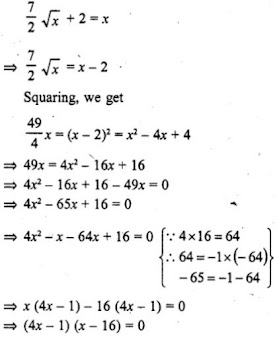x(4x-1) - 16(4x-1) = 0
⇒ (4x-1) (x-16) = 0
Either 4x-1 = 0, then
4x = 1
⇒ x = 1/4
but it is not possible being in fraction.
or x-16 = 0, then x = 16
Thus, number of swans are 16.

5. If the list price of a toy is reduced by Rs. 2, a person can buy 2 toys more for Rs. 360. Find the original price of the toy.

Solution

List price of the toy = Rs. x
Total amount = Rs. 360
Reduced price of each toy = (x – 2)
According to the question,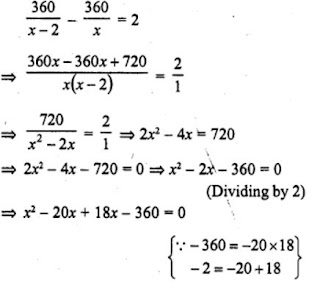⇒ x (x – 20) + 18 (x – 20) = 0
⇒ (x – 20) (x + 18) = 0
Either x – 20 = 0, then x = 20
or x + 18 = 0, then x = -18 which is not possible being negative
Price of each toy = Rs. 20

6. Rs 9000 were divided equally among a certain number of persons. Had there been 20 more persons, each would have got Rs. 160 less. Find the original number of persons.

Solution

Total amount = Rs. 9000
Let number of persons = x
Then each share = Rs. 9000/x
Increased persons = (x + 20)
According to the question,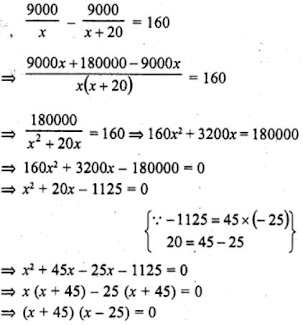Either x + 45 = 0, then x = -45 which is not possible being negative
or x – 25 = 0, then x = 25
Number of persons = 25

7. Some students planned a picnic. The budget for food was Rs. 500. But, 5 of them failed to go and thus the cost of food for each member increased by Rs. 5. How many students attended the picnic ?

Solution

Let number of students = x
Total budget = Rs. 500
Share of each student = Rs. 500/x
No. of students failed to go = 5
According to the given question,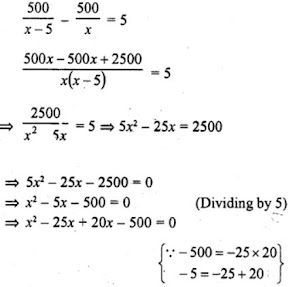⇒ x(x-25) + 20(x-25) = 0
⇒ (x-25) (x+20) = 0
Either x-25 = 0, then x = 25
or, x+20 = 0, then x = -20 which is not possible being negative.
Number of students = 25

8. A pole has to erected at a point on the boundary of a circular park of diameter 13 metres in such a way  that the difference of its distances from two diametrically opposite fixed gates A and B on the boundary is 7 metres. Is it the possible to do so ? If yes at what distance from the two gates should the pole be erected ?

Solution

In a circle, AB is the diameters and AB = 13 m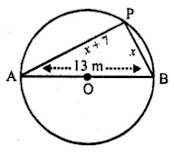Let P be the pole on the circle.

Let PB = x m, then PA = (x + 7) m

Now, in right ∆APB (P is in a semi circle)

AB2 = AB2 + AP2

⇒ (13)2 = x2 + (x+7)2

⇒ x2 + x2 + 14x + 49 = 169

⇒ 2x2 + 14x + 49 - 169 = 0

⇒ 2x2 + 14x - 120 = 0

⇒ x2 + 7x - 60 = 0 (Dividing by 2)

⇒ x2 + 12x - 5x - 60 = 0

⇒ x(x+12) - 5(x+12) = 0

⇒ (x+12) (x-5) = 0

Either x + 12 = 0, then x = -12 which is not possible being negative
or x – 5 = 0, then x = 5
P is at a distance of 5 m from B and 5 + 7 = 12 m from A.

9. In a class test, the sum of the marks obtained by P in Mathematics and science is 28. Had he got 3 marks more  in Mathematics and 4 marks less in science. The product of his marks, would have been 180. Find his marks in the two subjects.

Solution

Sum of marks in Mathematics and Science = 28
Let marks in Math = x
Then marks in Science = 28 – x
According to the question,
(x + 3) (28 – x – 4) = 180
⇒ (x + 3) (24 – x) = 180
⇒ 24x – x² + 72 – 3x = 180
⇒ 21x – x² + 72 – 180 = 0
⇒ – x² + 21x – 108 = 0
⇒ x² – 21x + 108 = 0
⇒ x² – 9x – 12x + 108 = 0
⇒ x (x – 9) – 12 (x – 9) – 0
⇒ (x – 9)(x – 12) = 0
Either x – 9 = 0, then x = 9
or x – 12 = 0, then x = 12
(i) If x = 9, then Marks in Maths = 9 and marks in Science = 28 – 9 = 19
(ii) If x = 12, then Marks in Maths = 12 and marks in Science = 28 – 12 = 16

10. In a class test, the sum of Shefali's marks in Mathematics and English in 30. Had she got 2 marks more in Mathematics and 3 marks less in English, the product of her marks would have been 210. Find her marks in two subjects.

Solution

Sum of marks in Mathematics and English = 30
Let marks obtained in Mathematics = x
Then in English = 30 – x
According to the question,
(x + 2) (30 – x – 3) = 210
⇒ (x + 2) (27 – x) = 210
⇒ 27x – x² + 54 – 2x – 210 = 0
⇒ – x² + 25x – 156 = 0
⇒ x² – 25x + 156 = 0
⇒ x² – 12x – 13x +156 = 0
⇒ x (x – 12) – 13 (x – 12) = 0
⇒ (x – 12) (x – 13) = 0
Either x – 12 = 0, then x = 12
or x – 13 = 0, then x = 13
(i) If x = 12, then
Marks in Maths =12 and in English = 30 – 12 = 18
(ii) If x = 13, then
Marks in Maths = 13 and in English = 30 – 13 = 17

11. A cottage industry produces a certain number of pottery articles in a day. It was observed on a particular day that the cost of production of each article (in rupees) was 3 more than twice the number of articles produced on that day. If the total cost of production on that day was Rs. 90, find the number of articles produced and the cost of each article.

Solution

Total cost = Rs. 90
Let number of articles = x
Then price of each articles = 2x + 3
x (2x + 3) = 90
⇒ 2x² + 3x – 90 = 0
⇒ 2x² – 12x + 15x – 90 = 0
⇒ 2x (x – 6) + 15 (x – 6) = 0
⇒ (x – 6) (2x + 15) = 0
Either x – 6 = 0, then x = 6
or 2x + 15 = 0 then 2x = -15
⇒ x = −15/2 which is not possible being negative
x = 6
Number of articles = 6
and price of each article = 2x + 3 = 2 x 6 + 3 = 12 + 3 = 15

At t minutes past 2 pm the time needed by the minutes hand and a clock to show 3 pm was found to be 3 minutes less than t2/4 minutes. Find t.
Solution

We know that, the time between 2 pm to 3 pm = 1 h = 60 minutes
Given that, at t minutes past 2 pm, the time needed by the min. hand of a clock to show 3 pm was found to be 3 min. less than t2/4 min.
i.e., t = (t2/4 – 3) = 60
⇒ 4t + t² – 12 = 240
⇒ t² + 4t – 252 = 0
⇒ t² + 18t – 14t – 252 = 0 [by splitting the middle term]
⇒ t (t + 18) – 14 (t + 18) = 0
⇒ (t + 18) (t – 14) = 0 [since, time cannot be negative, so t ≠ -18]
t = 14 min.
Hence, the required value of t is 14 minutes.